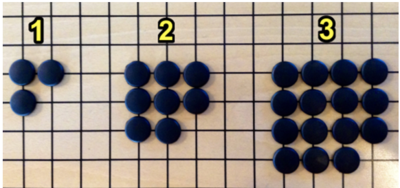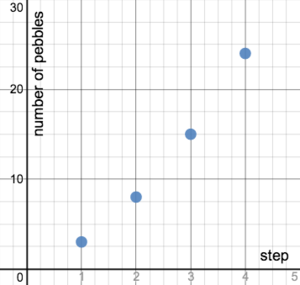## Alert!

Hello, reader! If you intend to post a link to this blog on Twitter, be aware that for utterly mysterious reasons, Twitter thinks this blog is spam, and will prevent you from linking to it. Here's a workaround: change the .com in the address to .ca. I call it the "Maple Leaf Loophole." And thanks for sharing!

## Saturday, August 16, 2014

### Is it linear?

Hi! This is a task I helped develop that uses concrete examples to help students notice the traits of a linear relationship in different representations in service of CCSS standard 8.EE.B. I'm publishing it here to make it available. (If you know of something better to achieve the same purpose, and is easily-accessible, please let me know!)

Below are several situations which describe a relationship between two quantities which are each noted in parentheses. Your goal is to determine which of these relationships is linear. To help you decide, consider creating a table of values to compare the quantities specified in parentheses, graphing the relationship on a coordinate plane, or writing an equation to represent the relationship. Make sure to define any variables clearly, with appropriate units.
1. The price of bananas at the Italian Market is \$2 for every 3 pounds (cost and pounds).
2. Tamika plants a tree that is 3 inches tall, and it grows 6 inches per month (height and months).
3. Rhys folded a strip of paper that is 120 cm long into halves over and over so that its length shrinks by half each time (length and number of folds).
4. The perimeter of an equilateral triangle is three times its side length (perimeter and side length).
5. Malcolm bikes for half an hour at 30 miles per hour, followed by an hour at 15 miles per hour. He rests for half an hour and then bikes at 20 miles an hour for 1.5 hours (distance and hours).
6. The area of a circle is π times its radius squared (area and radius).
7. A teacher plans to grade exams for a whole day, and estimates that she grades 8 exams every 30 minutes (number of tests that have been graded and minutes).
8. (Number of squares and step)9. (Number of pebbles vs. step)
10.What are some properties you used to determine whether a relationship is linear or non-linear?

### Commentary

This task uses concrete examples to help students notice the traits of linear relationships in different representations, and prepares them to see the connections between proportional relations, lines, and their equations for 8.EE.B. Since the given scenarios cover a wide range of contexts, what makes a relationship linear may not be easily discernable by reading the descriptions.  Translating them into tables, graphs, and equations enables students to spot and articulate regularity and structure in situations that produce line graphs (MP7, MP8) and to generalize these regularities as clues of linear relations.
Two of the scenarios (b and h) involve non-proportional, linear relationships. A teacher may opt to leave them out if she wants to focus on constant rate of change without the added wrinkle of accounting for a starting value.
As an instructional activity, there are many ways to implement this task. For example, a teacher could create cards showing an equation, table, and graph for each scenario, and turn it into a matching activity. This approach would decrease the level of difficulty substantially, but in skilled hands, could still provide fodder for a rich conversation about the features of a situation that imply a linear relationship.
Another approach might be to "jigsaw" the activity. One team of students might be responsible for creating tables, another graphs, and another equations. The teams could reconfigure to contain one student using each representation, and work together to see if their representations are consistent. They could write down some characteristics that tell you whether a situation is linear or non-linear, leading into a whole-class discussion.

## Solution

An equation is provided, when possible, to provide the teacher with succinct information about the relationship. Students may frame their arguments by creating tables or graphs and using them to support their reasoning, and some samples are provided. The important characteristic of a linear relation is for the quantity to exhibit a constant difference over consistent intervals.

a. Linear. Let c = cost in dollars and p = weight in pounds.
c=2p3, where p0

 p 0 3 6 9 c 0 2 4 6b. Linear. Let m = number of months and h = height in inches. h=6m+3 Note: since the tree will not keep growing at the same rate forever, this equation only works for reasonable values of m. Students may discuss how they might limit what values were "allowed" for m.

c. Not linear. Let n = number of folds and L = length in cm.
L=120(12)n where n can only be natural numbers (you can't fold a piece of paper 4/5 of a time, for example).

 n 0 1 2 3 L 120 60 30 15

d. Linear. Let P = perimeter in any units of length and s = side length in the same units.
P=3s or s=P3

 s 0 1 2 3 P 0 3 6 9

e. Not linear. At certain time intervals, his distance traveled is linear, but he does not have a constant rate of change for his whole trip.f. Not linear. Let r = radius in units and A = area in square units.
A=πr2 or r=Aπ
g. Linear. Let m = time in minutes and T = number of tests that have been graded.
T=8m30

 m 0 30 60 90 T 0 8 16 24

h. Linear. Let s = step and N = number of squares. N = 3s + 4, where s is a natural number.

 s 1 2 3 4 N 7 10 13 16

i. Not linear. Let s = step and P = number of pebbles.  P=(s+1)21 where s is a natural number.#### Post a Comment

Hi! I will have to approve this before it shows up. Cuz yo those spammers are crafty like ice is cold.